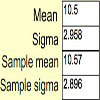#### You may also like### Spinners

How do scores on dice and factors of polynomials relate to each other?### Data Matching

Use your skill and judgement to match the sets of random data.### Random Inequalities

Can you build a distribution with the maximum theoretical spread?

# Time to Evolve 2

##### Age 16 to 18Challenge Level

The span of time between births of Parent and Child is [5, 15]. Similarly for Grandparent and Parent. Therefore, from Grandparent to Child is [5, 15] + [5, 15] = [10, 30].
The longest time is 30 years, the shortest is 10 years.

The uniform model has the advantage of having a simple pdf thus making analysis comparatively straightforward.
It is, however, an idealised model; in practice spans at the shorter end of the interval would be more likely, and there would not be sudden cut-offs at the 5 and 15 year marks. Also, the uniform pdf is defined piecewise which complicates the analysis in this question.
A more realistic model might be $T_2 \sim N(10, 25/3)$, which has the same mean and standard deviation as the uniform model but falls off more smoothly at the edges. However, this model allows values outside the range [5, 15], and even negative values, which are clearly invalid.

The p.d.f. of $T_3$ is $f(t) = \begin{cases}{{t-10}\over{100}} & {10\le t\le 20}\\ {{30-t}\over{100}} & {20\le t\le 30}\\{0} & {otherwise} \end{cases}$
Its graph is an isosceles triangle with vertices at (10, 0), (20, 0.1) and (30, 0).

$T_{10}$ is the sum of 9 independent $T_2$s,
$\sum_{i=1}^9\left(5+10U_i[0,1]\right)$
so
$T_{10}=45+ 10\sum_{i=1}^9\left(U_i[0,1]\right)$
While it is difficult to find the pdf of this distribution, we can find its mgf (moment generating function), as follows.
Let $U\sim U[0,1]$
Then $f_U(u)=\begin{cases}1 & 0\le u\le 1\\ 0 & otherwise\end{cases}$
The moment generating function $M_X(t)$ of a random variable X is defined as $M_X(t){\buildrel\rm def\over =} E(e^{tX})$
Hence
\begin{align} M_U(t) & = E(e^{tU}) \\ & = \int_{-\infty}^{\infty}{e^{tu}f_U(u)du} \\ & = \int_0^1{e^{tu}du} \\ & = \left [ e^{tu} \over t \right ]_0^1 \\ & = {{e^t-1} \over {t}} \end{align}
Let $S = \sum_{i=1}^n U_i$
It is an important fact about MGFs that where independent random variables are added, their MGFs multiply.
Hence, the MGF of S
$M_S(t) = \prod_{i=1}^n M_{U_i}(t)$
and since all the $M_{U_i}$ are the same,
$M_S(t) = {M_U(t)}^n = \left ({{e^t-1} \over t}\right )^n$

We now wish to use this mgf to calculate the mean and variance of S, using the following formulae:
$E(S) = {M_S^\prime}(0)$
$Var(S) = {M_S^{\prime\prime}}(0) - \{{{M_S^\prime}(0)}\}^2$
However, $M_S(t)$ is not defined for t=0. We can, however, get around this problem by writing $e^t$ as its Maclaurin series.
\begin{align}M_S(t) & = \left({{e^t-1}\over{t}}\right)^n \\ & = \left( {{(1+t+{{t^2}\over{2}}+{{t^3}\over{3!}}+\dots + {{t^r}\over{r!}}+\dots) - 1} \over {t}}\right)^n \\ & = \left( {t + {{t^2}\over{2}}+{{t^3}\over{3!}}+\dots +{{t^r}\over{r!}}+\dots} \over {t} \right)^n \\ & = \left( 1 +{t \over 2} + {{t^2}\over{3!}}+\dots+{{t^{r-1}}\over{r!}}+\dots \right)^n \end{align}
Note that such term-by-term operations as the above division are valid only when the series satisfies certain convergence conditions.
We can now differentiate $M_S$ to give the mean and variance:
\begin{align}{M_S^\prime}(t) & = {{d}\over{dt}} \left( 1 +{t\over 2} + {{t^2}\over{3!}}+\dots+{{t^{r-1}}\over{r!}}+\dots \right)^n \\ & = \frac{d}{du}(u^n) \cdot \frac{du}{dt}\; \mbox{where}\; u=1 + {t\over 2}+{{t^2}\over{3!}}+\dots+{{t^{r-1}}\over{r!}}+\dots \\ & = n\left( 1 + {t\over 2} + {{t^2}\over{3!}}+\dots+{{t^{r-1}}\over{r!}}+\dots \right)^{n-1} \cdot \left( {1\over 2} + {{2t}\over{3!}}+\dots+{{(r-1)t^{r-2}}\over{r!}}+\dots \right)\end{align}
Therefore ${M_S^\prime}(0) = n \cdot {1\over 2} = {n\over 2}$
Hence $E(S) ={n\over 2}$
A further differentiation gives ${M_S^{\prime\prime}}(0) = \frac{n^2}{4} + \frac{n}{12}$
and hence $Var(S) = \frac{n^2}{4} + \frac{n}{12} - \left(\frac{n}{2}\right)^2 = \frac{n}{12}$

$T_{10} = 45 + 10 S_9$, so $E(T_{10}) = 45 + 10 \cdot \frac{9}{2} = 90$ and $Var(T_{10}) = 10^2 \cdot \frac{3}{4} = 75$

For large values of n, typically $n \ge 30$, the Central Limit Theorem states that the mean of a sample drawn from a continuous distribution, regardless of the shape of the distribution from which it was taken, will be distributed approximately normally. This implies that the sum is also distributed approximately normally, and hence, for $n \ge 30$,
$T_{n} \approx N(5(n-1) + 10\cdot\frac{n-1}{2}, 10^2\cdot\frac{n-1}{12})$
$\Rightarrow T_{n} \approx N(10(n-1), \frac{25(n-1)}{3})$